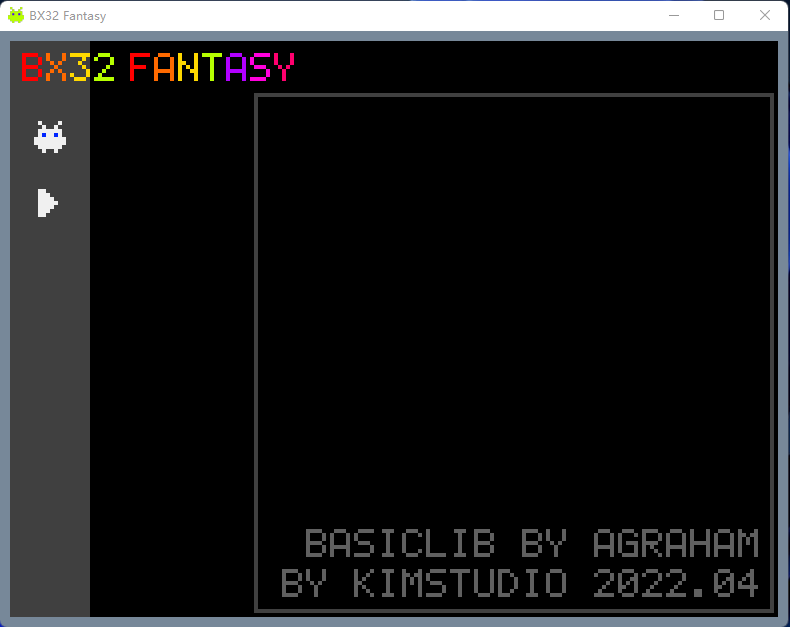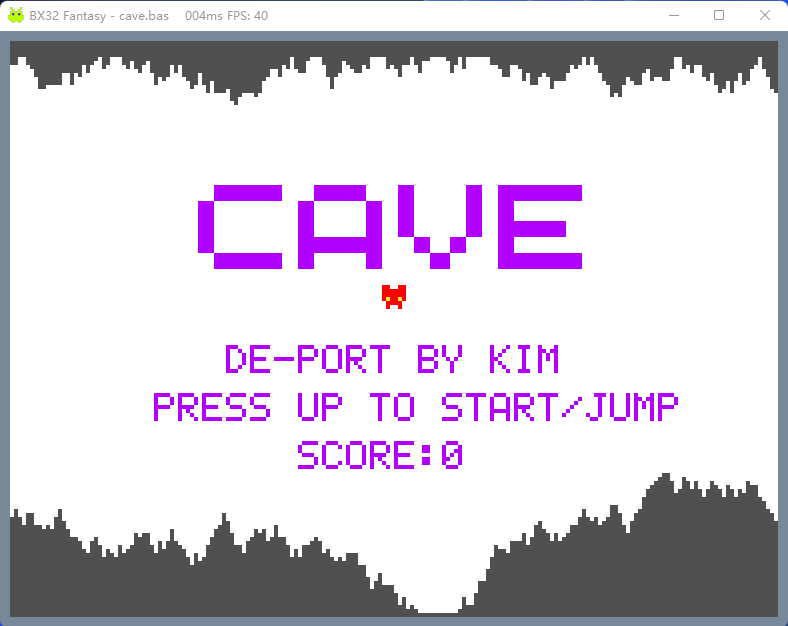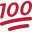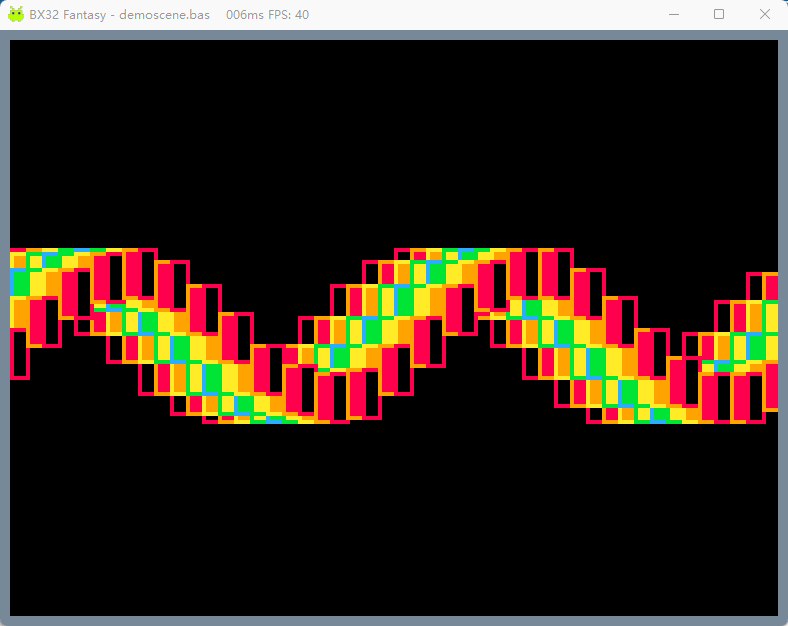# Share My CreationBX32 Fantasy Player

BX32 is a initial version of fantasy player to create and play tiny games in BASIC language. After investigated PICO8/TIC80 and found we have BasicLab to run basic script made by @agraham, I am trying to implement some functionalities of a fantasy computer. Usually the fantasy computer includes a complete IDE to edit script, sprite, map and sound, but I realize it is too time consuming and it is more convinient to use 3rd party tools, although I think map and sound editor should be included.

Specification:
screen size: 192 * 144
sprite sheet: 128*128 png file with full colors. sprite size 8*8
sound: mp3, wav, ...
control: keyboard: up, down, left, right, z, x

API:
bx_rgb(r,g,b): return color made of r, g, b
bx_cls(color): draw background using color
bx_tset(color): set color as transparent color when draw sprite
bx_camera: not used (I am not sure how camera works for all drawings or only for sprite..)
bx_clip(x0,y0,x1,y1): clip area when draw
bx_clipr: reset clip to full screen
bx_pset(x,y,color): draw a pixel at x, y using color
bx_pget(x,y): return pixel color at x, y
bx_sset(x,y,color): set pixel color at x, y in sprite sheet
bx_sget(x,y): get pixel color at x, y in sprite sheet
bx_palspron(0 or 1): set sprite in palette mode, in this mode the color will be palette index, palette has 16 colors with index 0-15
bx_palsprset(i, color): set palette index i to color
bx_palsprget(i): get color of palette index i
bx_palsprr: reset palette colors to default
bx_tpalsprset(i): use color at index i in palette as transparent color
bx_text(s, x, y, color): print text s at x, y using color. font is 5*7, only ascii
bx_spr(i, x, y): draw a sprite with its index i at x, y. sprite sheet is 128*128 so index is from top, left to bottom, right with 8*8 sprite size
bx_sprx(sx, sy, x, y, w, h): copy sprite sheet from x, y with w, h to screen sx, sy
bx_line(x0, y0, x1, y1, color): draw line
bx_lineh(x0, x1,y0, color): draw horizontal line using color
bx_linev(x0, y0, y1, color): draw vertical line using color
bx_rect(x0, y0, x1, y1, color): draw rectangle using color
bx_rectfill(x0, y0, x1, y1, color): fill rectangle using color
bx_circ((x, y, r, color): draw circle
bx_circfill(x, y, r, color): fill circle
bx_btn(i): return true or false if button pressed, 0 - 5 = left, right, up, down, z, x
bx_btnp(i): return true or false if button released, useful for menu control
bx_mousex: return mouse x pos
bx_mousey: return mouse y pos
bx_sfxset(i, soundname): set sfx sound file at id i. sound file must be at same directory with code *.bas
bx_sfx(i): play sfx sound of id i
bx_rnd(i, j): return int random number [i, j)

sub update
# any logic update code
end sub

sub draw
# any draw code
end sub

All API's must use int as parameters if it needs a number. FPS is locked at 40. The update and draw sub must be in code script which be called each frame.

Currently it supports to load a script file with .bas, and a .png for sprite, they must have same filename. sfx/sound mp3 files can have any filenames.
For the CAVE example, I have cave.bas, cave.png, cavesfx1.mp3 and cavesfx2.mp3 in same directory. Click the elf button to load cave.bas, then the cave.png will show on right. Click play button to run. Click Escape key to return to main console. If any errors in the bas code, edit it in an editor you like, save it, then click play again. It could be only with a bas code file.

To learn the basic script language need to refer to B4script.chm file posted by agraham.Attachements: BX32.jar (java version "1.8.0_202", java -jar BX32.jar); demos: cave, 3dplot, static tv scripts

cave code:
``````# cave
# de-port by kimstudio for BX32 from https://www.lexaloffle.com/bbs/?pid=105430#p

Dim Type(x, y, dy, rise, fall, dead, speed, score, jump_velocity) player
Dim caveu(192)
Dim caved(192)

textcolor = bx_rgb(178,0,255)
cavecolor = bx_rgb(80, 80, 80)
top = 60
btm = 144-60
gravity=0.2
game_over = true
speed_step = 1
point_step = 1500
next_step = point_step

bx_sfxset("cavesfx1.mp3", 1)
bx_sfxset("cavesfx2.mp3", 2)

make_player
make_cave

sub make_player
player.x=40
player.y=72
player.dy=0
player.rise=1
player.fall=2
player.speed=2
player.score=0
player.jump_velocity=3
end sub

sub draw_player
if game_over then
else if(player.dy < 0) then
bx_spr(player.rise,player.x,player.y)
else
bx_spr(player.fall, player.x, player.y)
end if
end sub

sub move_player
player.dy = player.dy + gravity
if bx_btnp(2) then
bx_sfx(1)
player.dy = 0
player.dy = player.dy - player.jump_velocity
end if
player.y = player.y+player.dy
player.score = player.score+int(player.speed)

end sub

sub check_hit
for i=player.x to player.x+7
if (caveu(i+1)>player.y or caved(i+1)<player.y+7) then
game_over=true
bx_sfx(2)
end if
next
end sub

sub speed_modifier
if (next_step < player.score) then
player.speed = player.speed + speed_step
next_step = player.score + point_step
point_step = point_step + point_step / 2
end if
end sub
# ---------------------------------
sub mid(a, b, c)
m = a
if a < b then
if a < c then
if b < c then
m = b
else
m = c
end if
else
m = a
end if
else
if b < c then
if a < c then
m = a
else
m = c
end if
else
m = b
end if
end if
return m
end sub

sub make_cave
caveu(0) = 5
caved(0) = 119
for i = 1 to 191
up = int(bx_rnd(0,7)-3)
dwn = int(bx_rnd(0,7)-3)
caveu(i) = mid(3,caveu(i-1)+up,top)
caved(i) = mid(btm,caved(i-1)+dwn,144)
next
end sub

sub update_cave
for i= 0 to 191-player.speed
caveu(i) = caveu(i+player.speed)
caved(i) = caved(i+player.speed)
next

for i = 191-player.speed to 191
up = int(bx_rnd(0,7)-3)
dwn = int(bx_rnd(0,7)-3)
caveu(i) = mid(3,caveu(i-1)+up,top)
caved(i) = mid(btm,caved(i-1)+dwn,144)
next
end sub

sub draw_cave
for i= 0 to 191
bx_line(i, 0, i, caveu(i), cavecolor)
bx_line(i,143,i, caved(i), cavecolor)
next
end sub
# ---------------------------------
sub draw_cover
draw_cave
bx_sprx(46,35,0,105,98,23)
bx_text("DE-PORT BY KIM", 54, 76, textcolor)
bx_text("PRESS UP TO START/JUMP", 36, 88, textcolor)
bx_spr(player.fall, 92, 60)
bx_text("SCORE:" & player.score, 72, 100, textcolor)
end sub
# ---------------------------------
sub update
if not(game_over) then
update_cave
move_player
check_hit
speed_modifier
else
if bx_btnp(2) then
game_over = false
make_player
end if
end if
end sub

sub draw
bx_cls(bx_rgb(255,255,255))
if game_over then
draw_cave
draw_cover
else
draw_cave
draw_player
bx_text("SCORE:" & player.score, 1, 133, textcolor)
end if
end sub``````

#### Attachments

• BX32Demos.zip
19.8 KB · Views: 79
• BX32.jar
415.6 KB · Views: 51
Last edited:
•••Sandman, Jmu5667, micro and 11 others

#### kimstudio

##### Active Member
Longtime User
Correction:
bx_sfxset(soundname, i): set sfx sound file at id i. sound file must be at same directory with code *.bas

Another small demo: press z to change modedemo:
``````# demo for bx32 by kimstudio : porting from
#-- title:   Pretty Sphere, Pretty Spirals
#-- author:  xmonkee
#-- title: wave
#-- author: potato imaginator

mode = 0
phi=(1+sqrt(5))/2
ox=192/2
oy=144/2
t=0
n=888

cb = bx_rgb(0,0,0)

dim cpal(16)
for k = 0 to 15
cpal(k) = bx_palsprget(k)
next

Array(col, 8,9,10,11,12,11,10,9)
Array(col2, 0,8,9,10,11,10,9,8)

sub draw0
bx_cls(cb)
t=t+1
for i=1 to n
r=sin(sqrt(i/n)*2*cPI-t/128)*oy
th=2*cPI*(i/phi)+t/256
x=int(r*cos(th))
y=int(r*sin(th))
#bx_rgb(i%255,60,180)
bx_pset(x+ox,y+oy, bx_rgb(i%255,60,180))
next
end sub

sub draw1
bx_cls(cb)
t=t+1
for i=1 to n
r=sqrt(i/n)*oy
th=2*cPI*(i/phi)+t/128
x=int(ox+r*cos(th))
y=int(oy+r*sin(th))
bx_pset(x,y,bx_rgb(int(sin(r/oy-t/128)*255),60,180))
next
end sub

sub draw2
bx_cls(cb)
for i=-10 to 29
for j=0 to 8
x0 = 8*(i+j/2)
y0 = 68+16*cos(i/2+2*j/4+t)
x1 = x0 + 4
y1 = y0 + 12
bx_rectfill(x0,y0,x1,y1,cpal(col2(j%8)))
bx_rect(x0,y0,x1,y1,cpal(col(j%8)))
next
next
t=t-1/20
end sub

#----
sub update

end sub

sub draw
if bx_btnp(4) or bx_btnp(5) then
mode = mode + 1
t = 0
if mode = 3 then
mode = 0
end if
end if
if mode = 0 then
draw0
else if mode = 1 then
draw1
else
draw2
end if

end sub``````

••Jmu5667, micro, Johan Hormaza and 4 others